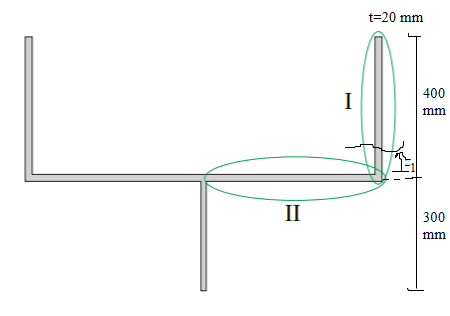# Find an expression for the shear stress of this figure

naji0044
Thread moved from the technical forums, so no Template is shown.
Summary: I have trouble to define the y distance in my coordinatesystemI need to find an expression for the shear stress on the I figure, where the green parabola illustrates the function. I made a cut, where I defined the length from the start of figure I to the cut as z1. The formula for calculating the shear stress:
τ=V*Q/I*t
V=183 kN (Shear)
Q=A*y
I=804.95*10^6 mm^4 (inertiamoment)
t=20 mm (thickness)

Ignore the II figure. My problem is on Q=A*y

The area A of the figure on my analyze is:
A=t*(400-z1)

The y distance confuses me and from the assignment, the center of the gravity is y=368.44 mm. So my thought is that the distance from the center of the figure I and the y=368.44 mm is expressed:
y=(400mm/2 - z1 - 368.44 mm)

So my function of shear stress is:

τ=183*10^3N* (t*(400-z1)) * (400mm/2 - z1 - 368.44 mm) / I * t

I think my y distance is wrong so would appreciate if someone could explain me how it is suppose to be.

## Answers and Replies

•